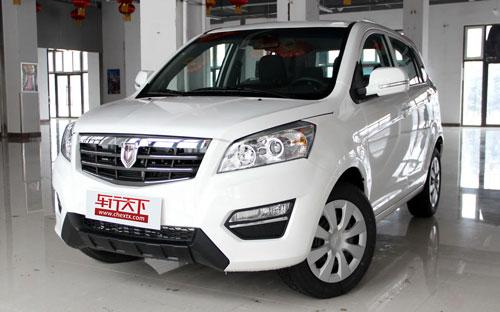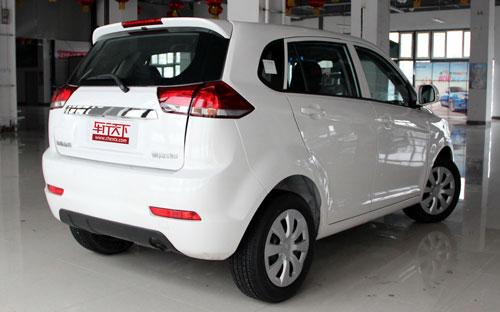绵阳金杯 智尚S306 种颜色可选2015款最低售价：4.98 万元起

4005(mm)1725(mm)1640(mm)配置亮点：
• 胎压监测装置

• ISOFIX儿童座椅接口

• 车身稳定控制(ESC/ESP/DSC等)

• 电动天窗

• 定速巡航

• 后倒车雷达

• 真皮座椅

• GPS导航系统

• 氙气大灯

• 后视镜加热

2015款 1.5L 手动电商版 (67张)绵阳金杯 智尚S30 在售车型

排量 车型 厂商指导价 本地最低报价 购车工具
1.5L 1.5L 自动豪华型 5挡AMT 6.98万 6.98万

询底价+对比
1.5L 自动精英型 5挡AMT 7.28万 7.28万

询底价+对比
1.5L 手动豪华型 5挡手动 6.58万 6.58万

询底价+对比
1.5L 手动精英型 5挡手动 6.88万 6.88万

询底价+对比
1.5L 自动舒适型 5挡AMT 5.98万 5.98万

询底价+对比
1.5L 手动电商版 5挡手动 4.98万 4.98万

询底价+对比
1.5L 手动舒适型 5挡手动 6.08万 6.08万

询底价+对比

绵阳金杯 智尚S30 经销商

查看更多 >>
更多 >>

本地降价排名

绵阳金杯 智尚S30 动力加速

智尚S30 0-100公里加速时间分布在 0.0-秒 属于 超跑级

动力级别 加速时间 车型

绵阳金杯 智尚S30 视频

绵阳金杯 智尚S30 新闻资讯

金杯S30上海车展静态评测 自主SUV又一新作

试驾评测 超过4213次关注

近几年自主品牌的发展非常迅猛，自主车型越来越成熟，它们也得到越来越多消费者的认可，一些原本在商用车中的品牌也加入到自主乘用车领域，例如江淮推出的和悦等车...

试驾金杯智尚S30 价格亲民的小型SUV

评测 超过3723次关注

说智尚S30，您不一定听过，但说金杯，这如雷贯耳的名字您肯定听过。今天文章的主角——华晨金杯智尚S30就是继S50之后，金杯旗下的第二款SUV，它个头很小，样子却很...

6万元左右小型SUV推荐 触手可及的SUV

导购 超过2147次关注

在印象中，金杯汽车一直是在做商用车，事实确实是这样，金杯进入乘用车市场还不久，目前的车型也很少。但这不妨碍我们推荐金杯智尚S30，这款产品保持了金杯汽车一贯...

金杯智尚S30自动挡上市 售6.88万元起

新闻 超过2477次关注

华晨汽车宣布向市场推出金杯智尚S30的AMT自动挡车型，共有两款不同配置车款，价格分别为6.88万和7.18万元。

预计7万左右 金杯智尚S30自动挡将上市

新闻 超过2417次关注

日前，我们得到消息，华晨汽车即将推出金杯智尚S30的自动挡车型，目前，金杯智尚S30三款手动挡车型的价格区间为5.98-6.78万元，据推测，其自动挡车型的价格可能在7...

金杯2014款智尚S30上市 售5.98-6.78万

新闻 超过2079次关注

近日我们从华晨官方获悉，金杯2014款智尚S30正式上市，三款车型的售价区间为5.98万-6.78万元。新车除了外形方面有一些改进之外，还对配置进行了一些升级。

猜你喜欢

﻿
• 快速找车
• 选择品牌
• 选择品牌
• A  奥迪
• A  阿斯顿·马丁
• A  阿尔法·罗密欧
• B  宝沃
• B  布加迪
• B  巴博斯
• B  保时捷
• B  宾利
• B  奔驰
• B  宝马
• B  本田
• B  别克
• B  标致
• B  比亚迪
• B  宝骏
• B  北汽制造
• B  北汽新能源
• B  北汽幻速
• B  北汽威旺
• B  北京汽车
• B  奔腾
• B  北汽绅宝
• C  长安
• C  长安商用
• C  长城
• C  昌河
• D  大众
• D  道奇
• D  DS
• D  东南
• D  东风风神
• D  东风风行
• D  东风小康
• D  东风风度
• D  东风
• F  福特
• F  丰田
• F  菲亚特
• F  法拉利
• F  福田
• F  福迪
• F  福汽启腾
• G  观致
• G  广汽传祺
• G  广汽吉奥
• G  GMC
• H  红旗
• H  汉腾汽车
• H  哈弗
• H  哈飞
• H  海格
• H  海马
• H  华颂
• H  黄海
• H  华泰
• H  恒天
• J  吉利汽车
• J  捷豹
• J  Jeep
• J  江淮
• J  江铃
• J  金杯
• J  九龙
• J  金旅
• K  凯翼
• K  凯迪拉克
• K  克莱斯勒
• K  科尼塞克
• K  卡威
• K  开瑞
• L  路虎
• L  林肯
• L  劳斯莱斯
• L  兰博基尼
• L  雷克萨斯
• L  铃木
• L  雷诺
• L  理念
• L  力帆
• L  莲花汽车
• L  猎豹
• L  路特斯
• L  陆风
• M  马自达
• M  MG
• M  MINI
• M  玛莎拉蒂
• M  摩根
• M  迈凯轮
• N  纳智捷
• O  欧宝
• O  讴歌
• O  欧朗
• Q  奇瑞
• Q  起亚
• Q  启辰
• R  日产
• R  荣威
• R  瑞麒
• S  三菱
• S  斯威汽车
• S  萨博
• S  smart
• S  斯柯达
• S  斯巴鲁
• S  思铭
• S  双龙
• S  上汽大通
• S  双环
• T  特斯拉
• T  腾势
• W  沃尔沃
• W  五菱汽车
• W  五十铃
• W  威兹曼
• W  威麟
• X  现代
• X  雪佛兰
• X  雪铁龙
• X  西雅特
• Y  一汽
• Y  英菲尼迪
• Y  英致
• Y  依维柯
• Y  野马汽车
• Y  永源
• Z  众泰
• Z  中华
• Z  中兴
• Z  知豆
• 选择车系
• 选择车系
• 车型对比
• 选择品牌
• 选择品牌
• A  奥迪
• A  阿斯顿·马丁
• A  阿尔法·罗密欧
• B  宝沃
• B  布加迪
• B  巴博斯
• B  保时捷
• B  宾利
• B  奔驰
• B  宝马
• B  本田
• B  别克
• B  标致
• B  比亚迪
• B  宝骏
• B  北汽制造
• B  北汽新能源
• B  北汽幻速
• B  北汽威旺
• B  北京汽车
• B  奔腾
• B  北汽绅宝
• C  长安
• C  长安商用
• C  长城
• C  昌河
• D  大众
• D  道奇
• D  DS
• D  东南
• D  东风风神
• D  东风风行
• D  东风小康
• D  东风风度
• D  东风
• F  福特
• F  丰田
• F  菲亚特
• F  法拉利
• F  福田
• F  福迪
• F  福汽启腾
• G  观致
• G  广汽传祺
• G  广汽吉奥
• G  GMC
• H  红旗
• H  汉腾汽车
• H  哈弗
• H  哈飞
• H  海格
• H  海马
• H  华颂
• H  黄海
• H  华泰
• H  恒天
• J  吉利汽车
• J  捷豹
• J  Jeep
• J  江淮
• J  江铃
• J  金杯
• J  九龙
• J  金旅
• K  凯翼
• K  凯迪拉克
• K  克莱斯勒
• K  科尼塞克
• K  卡威
• K  开瑞
• L  路虎
• L  林肯
• L  劳斯莱斯
• L  兰博基尼
• L  雷克萨斯
• L  铃木
• L  雷诺
• L  理念
• L  力帆
• L  莲花汽车
• L  猎豹
• L  路特斯
• L  陆风
• M  马自达
• M  MG
• M  MINI
• M  玛莎拉蒂
• M  摩根
• M  迈凯轮
• N  纳智捷
• O  欧宝
• O  讴歌
• O  欧朗
• Q  奇瑞
• Q  起亚
• Q  启辰
• R  日产
• R  荣威
• R  瑞麒
• S  三菱
• S  斯威汽车
• S  萨博
• S  smart
• S  斯柯达
• S  斯巴鲁
• S  思铭
• S  双龙
• S  上汽大通
• S  双环
• T  特斯拉
• T  腾势
• W  沃尔沃
• W  五菱汽车
• W  五十铃
• W  威兹曼
• W  威麟
• X  现代
• X  雪佛兰
• X  雪铁龙
• X  西雅特
• Y  一汽
• Y  英菲尼迪
• Y  英致
• Y  依维柯
• Y  野马汽车
• Y  永源
• Z  众泰
• Z  中华
• Z  中兴
• Z  知豆
• 选择车系
• 选择车系
• 选择车型
• 选择车型
• 意见反馈